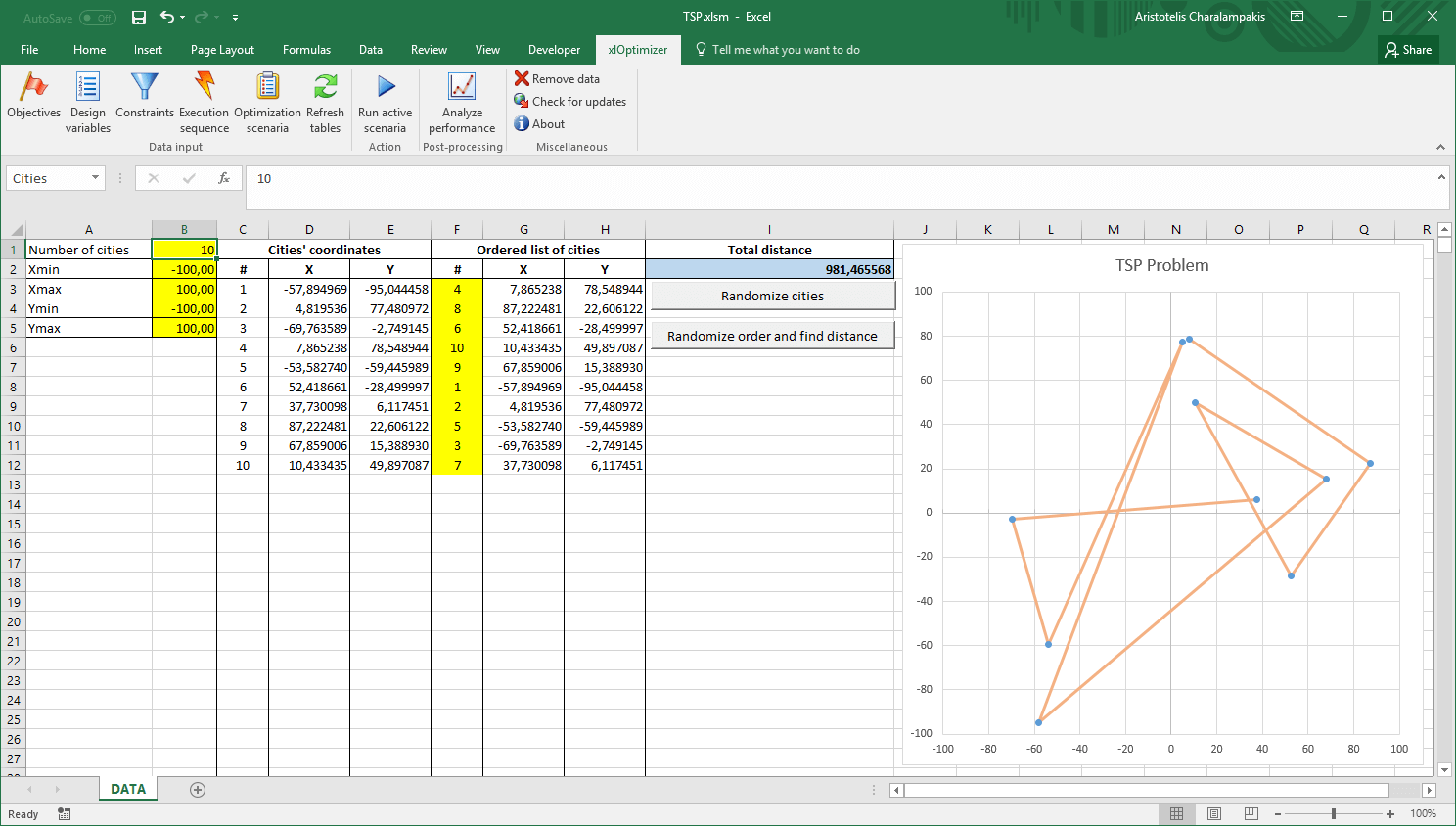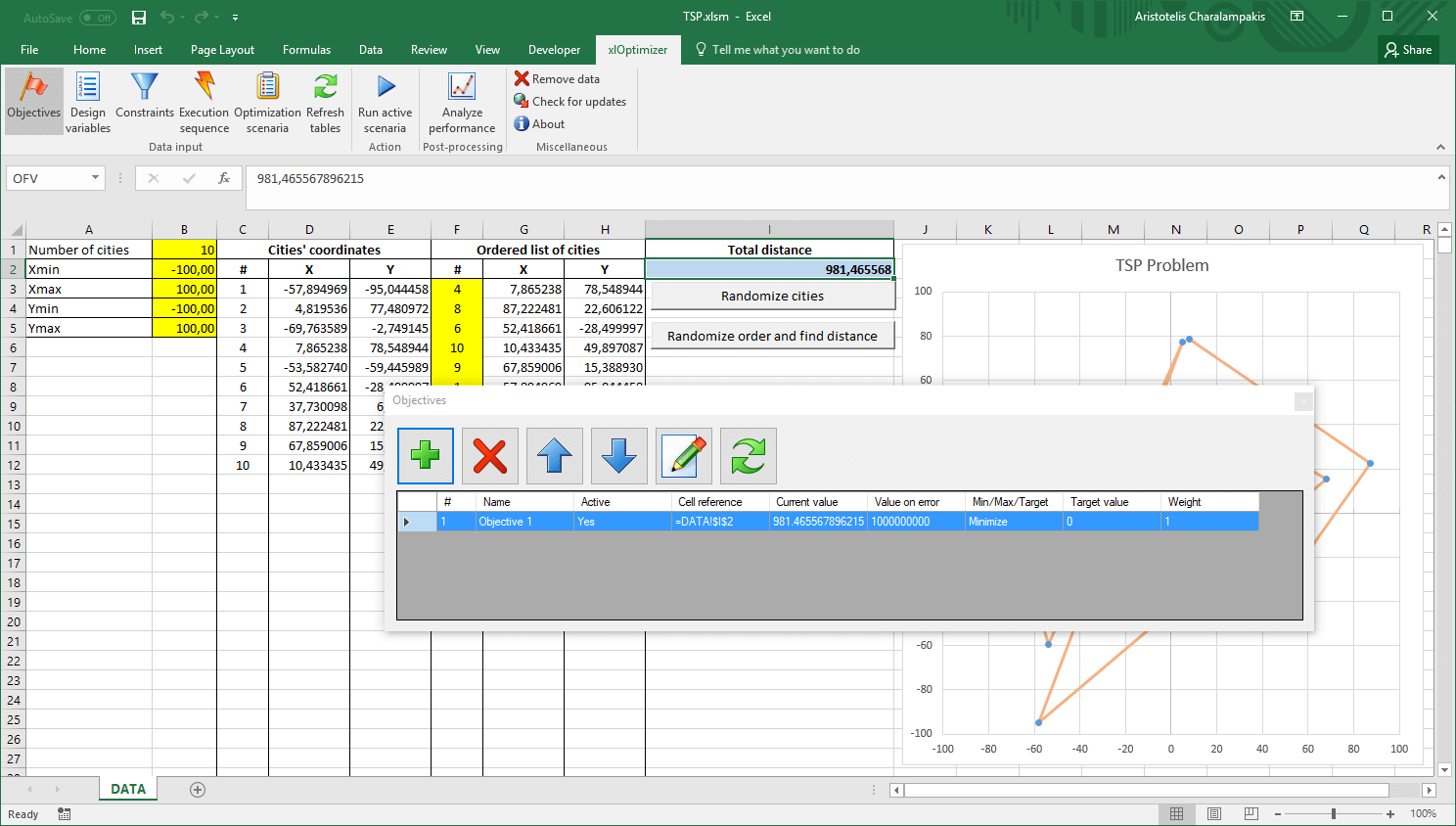## Introduction

The purpose of this article is to demonstrate the solution of the Travelling salesman problem (TSP) using metaheuristics in Microsoft Excel. The problem can be stated as follows:

"Given a list of cities and the distances between each pair of cities, what is the shortest possible route that visits each city exactly once?"

This is a well-studied NP-hard problem in combinatorial optimization. If N is the number of cities, a possible solution is given as a permutation of 1 to N that indicates the order in which the cities must be visited. To solve it, the generic Genetic Algorithm for permutation problems (PermGA) of xlOptimizer will be employed.

This article assumes that you have read and understood the procedures described in the toy problems. These include the optimization using pure Microsoft Excel formulas and the optimization using VBA (Visual Basic for Applications).

## Step 1 : Setting-up the spreadsheet

We have already created the spreadsheet for you. Although it is set-up for 10 cities (so that it can be used with the demo version of xlOptimizer add-in), you can change the number of cities easily by modifying cell B1 and pressing the Randomize cities button. Column F holds the current solution, i.e. the order in which the cities must be visited.

The evaluation of the total distance for the currently selected route is performed by a custom VBA routine, i.e. Sheet1.UpdateXYDistance. The code can be inspected if you produce the VBA editor (by pressing Alt+F11). Note that the implementation does not include a return trip from the last city to the first one, but the code can be modified easily.## Step 2 : Setting up the objective function

Select cell I2. This holds our objective function, i.e. the total distance of the currently selected route. In the xlOptimizer ribbon, press Objectives to produce the list of objectives. Then press the Add button '+':The objective is set to minimization by default. Press the Objectives button in the ribbon again to hide the objectives list.

## Step 3 : Setting up the design variables

Select the yellow cells of column F. These hold our design variables, i.e. the order of the cities.

In the xlOptimizer ribbon, press Design variables to produce the list of design variables. Then press the Add button '+' to add the cells to the list:There is no need to modify the properties of the design variables. By selecting PermGA, all cells will hold a permutation of integer numbers (1 to N, where N is the number of design variables). Press the Design variables button again in the ribbon to hide the design variable list.

## Step 4 : Setting up the constraints

In the present implementation, there are no constraints associated with the TSP problem. You can add any constraints you like by modifying the code and adding penalty to the objective function (i.e. artificially increase the total distance of the route), or by using the constraint list of xlOptimizer.

For example, a list of barrier lines, defined by the coordinates of their start and end points, can be set-up so that the route cannot cross them. This, however, exceeds the purpose of this article.

## Step 5 : Setting up the execution sequence

In the xlOptimizer ribbon, press Execution sequence to produce the list of execution commands. By default, a single Calculate command is listed. This is a call to Microsoft Excel's internal calculation routine, which evaluates the formulas such as '=SUM(C2:C6)'. Our custom routine handles everything, so we can substitute this with a macro call to routine Sheet1.UpdateXYDistance:Press Ok to save the changes. Press the Execution sequence button again in the ribbon to hide the execution sequence list.

## Step 6 : Setting up the optimization scenario

In the ribbon, press the Optimization scenaria button. Next, press the Add button '+' in the toolbar to add a new scenario. The following form appears:Select PermGA (permutation) as solver. This will assign permutations to all active design variables (integers from 1 to N). Also, selecting Order crossover method seems to work better with TSP. Make the appropriate selections and press Ok. The scenario is added to the list.

The data input is now complete. In the ribbon, press the Optimization scenaria button again to hide the list.

## Step 7 : Optimization

We can now proceed to the optimization by pressing the Run active scenaria button.

The file for this example can be downloaded here. Note that it is saved a macro enabled book with the extension .xlsm. You need to enable macros to run it.